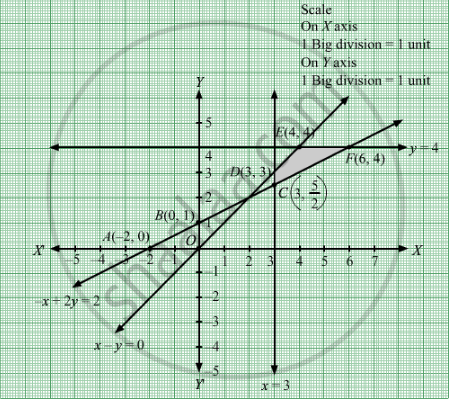# Minimize Z = X − 5y + 20 Subject to X − Y ≥ 0 − X + 2 Y ≥ 2 X ≥ 3 Y ≤ 4 X , Y ≥ 0 - Mathematics

Minimize Z = x − 5y + 20
Subject to

$x - y \geq 0$
$- x + 2y \geq 2$
$x \geq 3$
$y \leq 4$
$x, y \geq 0$

#### Solution

First, we will convert the given inequations into equations, we obtain the following equations:
x − y = 0, − x + 2y = 2, x = 3, y = 4, x = 0 and y = 0.

Region represented by x − y ≥ 0 or x ≥ y:
The line x − y = 0 or y passes through the origin.The region to the right of the line y will satisfy the given inequation.
Let's check by taking an example like if we take a point (4, 3) to the right of the line xy .Here ≥ y.So, it satisfy the given inequation.  Take a point (4, 5) to the left of the line x = y. Here, x ≤ y. That means it does not satisfy the given inequation.
Region represented by − x + 2y ≥ 2:
The line − x + 2y = 2 meets the coordinate axes at A(−2, 0) and B(0, 1) respectively. By joining these points we obtain the line
− x + 2y = 2.Clearly (0,0) does not satisfies the inequation − x + 2y ≥ 2. So,the region in xy plane which does not contain the origin represents the solution set of the inequation − x + 2y ≥ 2 .
The line x = 3 is the line that passes through the point (3, 0) and is parallel to Y axis. x ≥ 3 is the region to the right of the line x = 3.
The line y = 4 is the line that passes through the point (0, 4) and is parallel to X axis. y ≤ 4 is the region below the line y = 4.
Region represented by x ≥ 0 and y ≥ 0:
Since, every point in the first quadrant satisfies these inequations. So, the first quadrant is the region represented by the inequations x ≥ 0 and ≥ 0.
The feasible region determined by the system of constraints x − y ≥ 0,− x + 2y ≥ 2, x ≥ 3, ≤ 4, x ≥ 0 and y ≥ 0 are as follows.The corner points of the feasible region are $C\left( 3, \frac{5}{2} \right)$, $D\left( 3, 3 \right)$,  E(4, 4) and F(6, 4).
The values of Z at these corner points are as follows.

Corner point Z = x  − 5y + 20
$C\left( 3, \frac{5}{2} \right)$
3 − 5 × $\frac{5}{2}$+20= $\frac{21}{2}$

$D\left( 3, 3 \right)$
3 − 5 × 3 + 20 = 8
E(4, 4) 4 − 5 × 4 + 20 = 4
F(6, 4)
 6 − 5 × 4 + 20 = 6

Therefore, the minimum value of Z is 4 at the point E(4, 4). Hence, x = 4 and y = 4 is the optimal solution of the given LPP.
Thus, the optimal value of Z is 4.

Concept: Graphical Method of Solving Linear Programming Problems
Is there an error in this question or solution?

#### APPEARS IN

RD Sharma Class 12 Maths
Chapter 30 Linear programming
Exercise 30.2 | Q 14 | Page 32